## 【C++】C++ STL标准模板库知识点总结（秋招篇）

article2023/10/1 10:45:10/

### 文章目录

• 前言
• STL的六大组件是？
• 容器(container) 算法(algorithm) 迭代器(iterator) 三者的关系？
• 容器分为几种？分别有哪些？
• 关联性容器和非关联性容器有什么区别？
• Vector容器是怎么调整大小的？（内存不足时的操作）
• 迭代器和指针的区别
• 迭代器为何会失效呢？有何解决措施？
• 讲一下vector的erase函数 使用后迭代器的变化
• 其他容器（如list、deque、set、map等）的erase函数操作后，迭代器怎么变化的？
• Vector容器使用合集
• deque容器使用合集
• deque容器和Vector容器有何不同？
• 讲一讲deque容器的工作原理，缓冲区是什么，中控器又是什么？
• deque.begin()和deque.front(）效果一样吗
• string容器开辟的内存是连续的吗
• string容器使用合集
• 介绍一下STL中stack queue 它们代表什么数据结构
• 什么是适配器容器
• stack容器使用合集
• queue容器使用合集
• 介绍一下list容器，它是由哪种数据结构实现的？
• list容器使用合集
• 讲一下vector deque string list stack queue各自常用功能的时间复杂度
• STL的迭代器有几种
• vector deque string list stack queue set map用的什么迭代器?
• 讲一下RAII（Resource Acquisition Is Initialization）（资源获取即初始化）
• 介绍一下set容器，同时讲一下set和multiset的区别,
• set和multiset，与unorderedset有何区别？
• 讲讲红黑树 同时讲一下底层原理
• set容器使用合集
• 讲一下set容器和map容器的区别
• 为什么set容器里要考虑pair对组这个概念？
• set<>尖括号里可以放几个参数
• 讲一下map和mutimap的区别
• 如何判断map中哪个是键哪个是值；如何访问键和值？
• map，mutimap和unordered_map的区别
• map容器使用合集
• 讲一些STL中的back_inserter
• 讲一些STL中你常用的算法
• 成员函数push_back emplace_back的区别
• 讲一些STL中功能相似但不同的成员函数
• 讲一下 for_each transform两种遍历算法的区别
• 讲一下 find find_if adjacent_find binary_search count count_if等查找算法的区别
• 讲一下 sort random_shuffle merge reverse等排序算法的区别
• 讲一下 copy,replace,replace_if,swap等拷贝替换算法的区别
• 讲一下accumulate，fill等算术生成算法的区别
• 讲一下 set_intersection,set_union,set_difference等集合算法的区别
• 讲一下STL的空间配置器
• 以Vector为例，讲一下STL的空间配置器如何工作的
• 讲一下空间配置器的两层结构
• 讲一下内存池
• 讲一下仿函数
• 一元谓词和二元谓词的区别是什么？
• 内建函数对象是啥 ，和仿函数的关系？
• 介绍一下算术仿函数 关系仿函数 逻辑仿函数

# 前言

2023.7.28 首次更新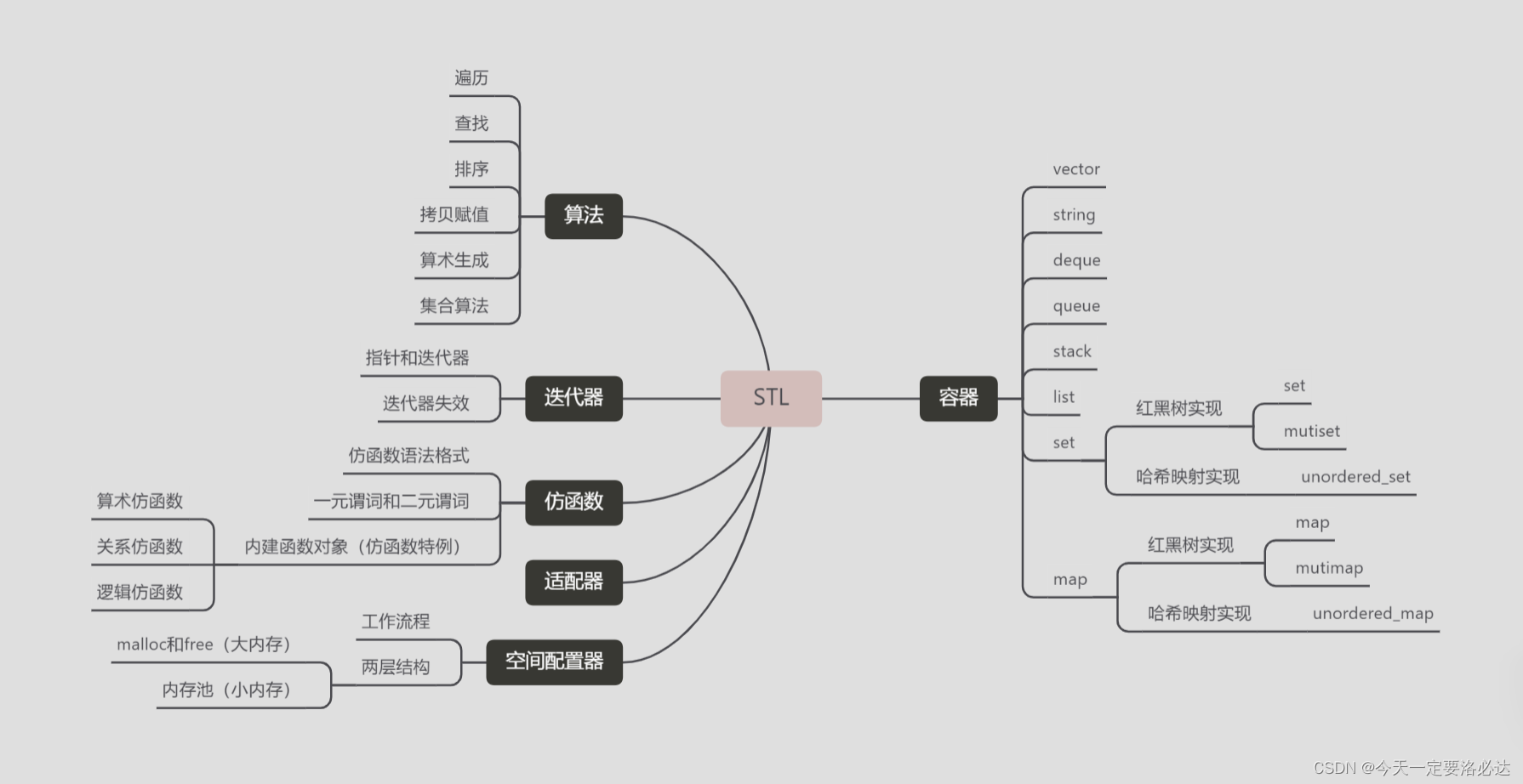## STL的六大组件是？

STL（标准模板库）是C++标准库中的一个重要组成部分，它提供了一套通用的模板类和算法，用于处理各种数据结构和操作。STL的六大组件如下：

1. 容器（Containers）：容器是用于存储和管理数据的类模板。STL提供了多种容器，包括向量（vector）、链表（list）、双端队列（deque）、集合（set）、映射（map）等。每种容器都有不同的特点和适用场景。
2. 算法（Algorithms）：算法是用于执行各种操作的函数模板。STL提供了大量的算法，例如排序、查找、合并、删除等。这些算法可以应用于各种容器，使得对数据的处理更加方便和高效。
3. 迭代器（Iterators）：迭代器用于遍历容器中的元素，类似于指针的概念。STL提供了多种类型的迭代器，包括输入迭代器、输出迭代器、前向迭代器、双向迭代器和随机访问迭代器。迭代器提供了统一的接口，使得算法可以独立于容器而操作。
4. 仿函数（Functors）：仿函数是重载了函数调用运算符的类对象，它可以像函数一样被调用。STL中的算法通常接受仿函数作为参数，用于指定特定的操作。STL提供了一些内置的仿函数，同时也可以自定义仿函数。
6. 分配器（Allocators）：分配器用于管理内存的分配和释放。STL提供了默认的分配器，同时也允许用户自定义分配器。分配器可以在容器中指定，用于控制容器的内存管理方式。

## 容器(container) 算法(algorithm) 迭代器(iterator) 三者的关系？

• 容器提供了数据的存储和组织方式，可以使用算法对容器中的数据进行操作。
• 算法是对容器进行操作的函数模板，可以独立于容器使用。
• 迭代器用于遍历容器中的元素，提供了访问容器元素的接口。

## 容器分为几种？分别有哪些？

1. `vector`：动态数组，支持快速随机访问。
2. `list`：双向链表，支持在任意位置进行插入和删除操作。
3. `deque`：双端队列，支持在两端进行插入和删除操作。
4. `array`：固定大小的数组，大小在编译时确定。
5. `forward_list`：单向链表，支持在任意位置进行插入和删除操作。

1. `set`：集合，存储唯一的元素，按照键的升序进行排序。
2. `map`：映射，存储键值对，按照键的升序进行排序。
3. `multiset`：多重集合，存储允许重复的元素，按照键的升序进行排序。
4. `multimap`：多重映射，存储允许重复的键值对，按照键的升序进行排序。

## Vector容器是怎么调整大小的？（内存不足时的操作）

1）如果我们要增加vector的大小，可以使用resize()函数或insert()函数来添加新的元素。

resize()函数：可以指定新的大小，并在尾部添加默认构造的元素。例如，myVector.resize(newSize);会将vector的大小调整为newSize，并在尾部添加默认构造的元素。

insert()函数：可以在指定位置插入元素，从而增加vector的大小。例如，myVector.insert(myIterator, element);会在myIterator指定的位置插入element元素。

2）如果我们要减小vector的大小，可以使用erase()函数来删除元素。

erase()函数：可以删除指定位置或指定范围内的元素，从而减小vector的大小。例如，myVector.erase(myIterator);会删除myIterator指定的位置上的元素。

3）如果我们要增加vector的大小，当新的大小超过当前容量时，vector会重新分配更大的内存空间。通常，vector会分配当前大小的两倍（或者更多）的内存空间，然后将原有的元素复制到新的内存空间中。

4）如果我们要减小vector的大小，当新的大小小于当前容量时，vector会将后续的元素向前移动来填补被删除元素的空缺。

1）概念上的区别：

2）灵活性和安全性：

3）容器的抽象：

4）迭代器分类：

## 迭代器为何会失效呢？有何解决措施？

1）插入和删除元素：当我们向容器中插入或删除元素时，可能会导致迭代器失效。插入元素可能会导致原有的迭代器指向的元素位置发生变化，删除元素可能会导致迭代器指向的元素被删除。

2）改变容器大小：当我们改变容器的大小（如调整vector的大小）时，可能会导致迭代器失效。改变容器大小可能会导致原有的迭代器指向的元素位置发生变化。

3）使用end迭代器：在使用迭代器遍历容器时，如果迭代器到达容器的end位置后继续使用，就会导致迭代器失效。end迭代器表示容器中最后一个元素的下一个位置，它不指向一个有效的元素。

1）在插入和删除元素时，尽量使用返回新的迭代器的插入和删除函数，而不是使用原有的迭代器。

2）在遍历容器时，使用前向迭代器或双向迭代器，避免使用随机访问迭代器。因为前向迭代器和双向迭代器的失效范围相对较小。

3）在改变容器大小之前，将需要保留的元素先拷贝到另一个容器中，然后重新构建原容器。

## 讲一下vector的erase函数 使用后迭代器的变化

erase()函数是STL容器提供的一个成员函数，用于从容器中删除一个或多个元素。它接受一个迭代器作为参数，指定要删除的元素的位置，并返回一个指向被删除元素之后元素的迭代器。

``````auto it = vec.erase(position);
``````

``````auto it = vec.erase(first, last);
``````

``````#include <iostream>
#include <vector>

int main() {
std::vector<int> vec = {1, 2, 3, 4, 5};

auto it = vec.erase(vec.begin() + 2); // 删除第三个元素

std::cout << "After erasing: ";
for (auto num : vec) {
std::cout << num << " ";
}
std::cout << std::endl;

std::cout << "Iterator position: " << *it << std::endl;

return 0;
}
``````

1）list：

2）deque：

3）set 和 map：

## Vector容器使用合集

``````#include<iostream>
#include<vector>
#include<algorithm>
using namespace std;
//自定义类
class person{
public:
person(int age,float high){
this->age=age;
this->high=high;
cout<<"对象建立成功！"<<endl;
}
void printself(){
cout<<"我的年龄是"<<this->age<<endl;
cout<<"我的身高是"<<this->high<<endl;
}
~person(){
cout<<"对象已经被销毁！"<<endl;
}
private:
int age;
float high;
};
//0.vector的三种遍历方式

//vector第三种遍历方式需要传入for_each的打印函数
void print3(int val){
cout<<val<<" ";
}
//vector遍历方式1
void printVector(vector<int> v){
vector<int>::iterator begin=v.begin();
vector<int>::iterator end=v.end();
while(begin!=end){
cout<<*begin<<" ";//相当于是解引用
begin++;
}
cout<<endl;
}
// vector遍历方式2(比较常用)
void printVector2(vector<int> v) {

for (vector<int>::iterator it = v.begin(); it != v.end(); it++) {
cout << *it << " ";
}
cout << endl;
}
// vector遍历方式3(使用STL提供的算法)
void printVector3(vector<int> v){
for_each(v.begin(),v.end(),print3);
cout<<endl;
}

int main(){

//vector基础操作指令集(以int为例)
//1.构造方式4种
vector<int> v1;//无参构造方式

for (int i = 0; i < 10; i++)
{
v1.push_back(i);
}
printVector(v1);
// printVector2(v1);
// printVector3(v1);
vector<int> v2(v1.begin(), v1.end());//使用迭代器范围构造
printVector(v2);

vector<int> v3(10, 100);//使用重载的构造函数构造
printVector(v3);

vector<int> v4(v3);//使用拷贝构造函数构造，属于深拷贝（vector的赋值就是深拷贝）
printVector(v4);

//2.赋值方式
vector<int> v5;
v5=v1;//直接赋值操作，也是深拷贝，和前面vector<int> v4(v3)比较像
//但是使用拷贝构造函数的方式更为直接和简洁，而赋值操作需要先创建空对象再进行赋值。

vector<int> v6;
v6.assign(v1.begin(), v1.end());//assign迭代器赋值方式

vector<int> v7;
v7.assign(10,100);//assign重载赋值方式
//3.容器和大小
if(v1.empty()){
cout<<"动态数组1为空！"<<endl;
}
else{
cout<<"动态数组1不为空！"<<endl;
cout<<"v1的容量是："<<v1.capacity()<<endl;//是动态变化的 比容器现在的大小大一些
cout<<"v1的大小是"<<v1.size()<<endl;//现在的大小
}
//4.插入和删除
v1.push_back(11);//尾插
v1.pop_back();//尾删
v1.insert(v1.begin(),100);//指定地方插入1个元素
v1.insert(v1.begin(),2,100);//指定地方插入多个元素
// printVector(v1);
v1.erase(v1.begin());//删除元素
// printVector(v1);
v1.erase(v1.begin(),v1.end());//清空方式1
v1.clear();//清空方式2
// if(v1.empty()){
//     cout<<"动态数组1为空！"<<endl;
// }

//5.数据存取
cout<<v1<<" ";//类似数组方法
cout<<v2.at(0)<<" ";//at方法
cout<<v3.front()<<" ";//取第一个元素
cout<<v4.back()<<" ";//取最后一个元素
cout<<endl;
//6.容器元素互换
cout<<"交换前"<<endl;
printVector(v5);
printVector(v3);
v5.swap(v3);
cout<<"交换后"<<endl;
printVector(v5);
printVector(v3);
//7.预留空间
v1.reserve(100000);//容器预留len个元素长度，预留位置不初始化，元素不可访问。

return 0;
}
``````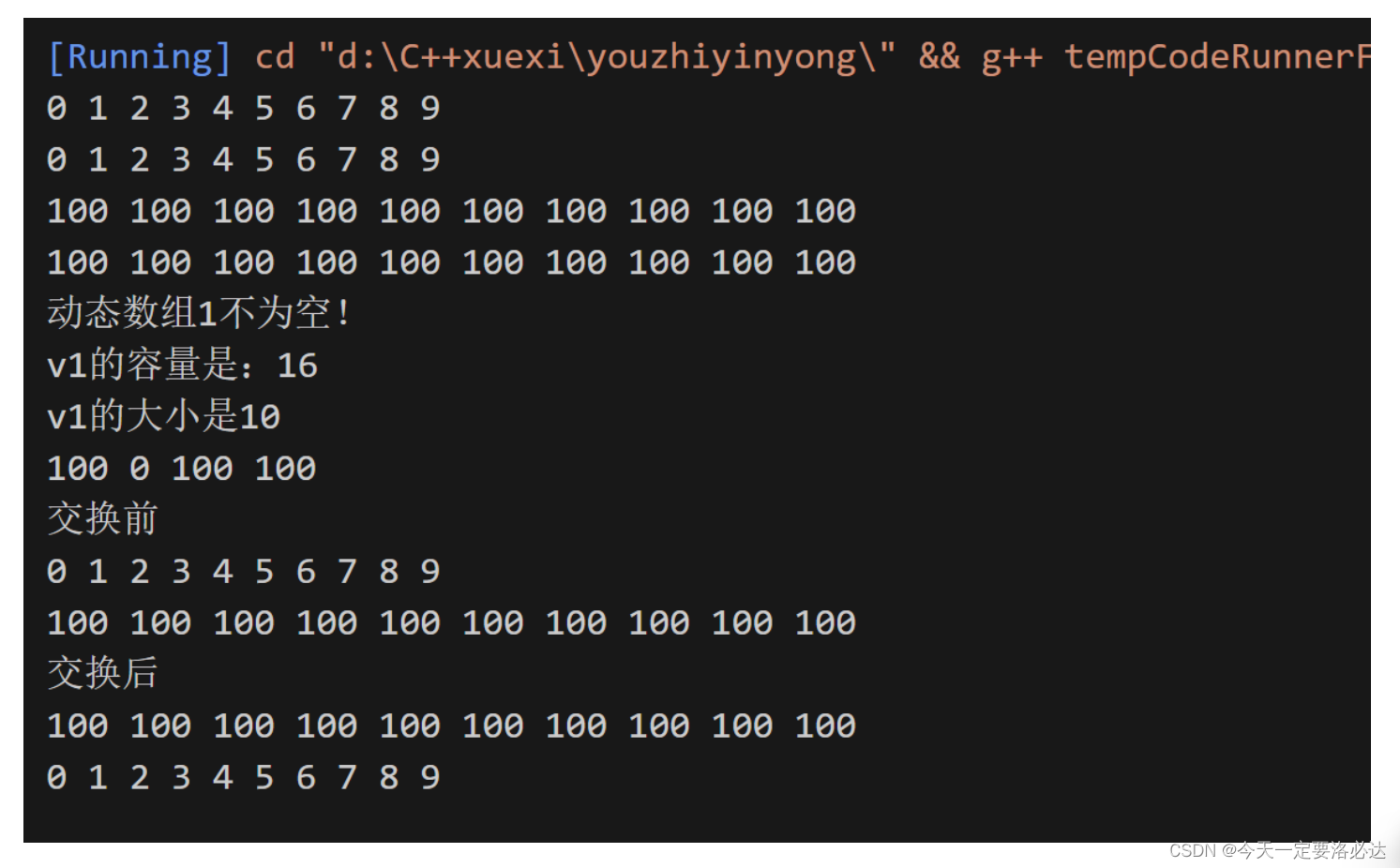## deque容器使用合集

``````#include <iostream>
#include <deque>
#include <algorithm>
using namespace std;

// 0.双端数组的遍历操作
void printDeque(const deque<int>& d)
//用const是为了防止改变传入对象的状态
//使用&是为了避免重复复制的开销
{
for (deque<int>::const_iterator it = d.begin(); it != d.end(); it++) {
cout << *it << " ";

}
cout << endl;
}

int main()
{
//deque基础操作指令集(以int为例)
//1.构造方式
deque<int> d1; //无参构造函数
for (int i = 0; i < 10; i++)
{
d1.push_back(i);
}
printDeque(d1);
deque<int> d2(d1.begin(),d1.end());//构造函数将[beg, end)区间中的元素拷贝给本身。
printDeque(d2);

deque<int>d3(10,100); //构造函数将n个elem拷贝给本身。
printDeque(d3);

deque<int>d4 = d3;//拷贝构造函数的一种形式
//另外一种deque<int>d4(d3)
printDeque(d4);

//2.赋值操作
deque<int> d21;
for (int i = 0; i < 10; i++)
{
d21.push_back(i);
}
printDeque(d21);

deque<int>d22;
d22 = d21; //重载等号操作符
printDeque(d22);

deque<int>d23;
d23.assign(d21.begin(), d21.end()); //将[beg, end)区间中的数据拷贝赋值给本身。
printDeque(d23);

deque<int>d24;
d24.assign(10, 100);//将n个elem拷贝赋值给本身。
printDeque(d24);

//3.容器大小操作
deque<int> d31;
for (int i = 0; i < 10; i++)
{
d31.push_back(i);
}
printDeque(d31);

//判断容器是否为空
if (d31.empty()) {
cout << "d31为空!" << endl;
}
else {
cout << "d31不为空!" << endl;
//统计大小
cout << "d31的大小为：" << d31.size() << endl;
}

//重新指定大小
d31.resize(15, 1);
//重新指定容器的长度为num,若容器变长，则以默认值填充新位置。
printDeque(d31);

d31.resize(5);
//重新指定容器的长度为num,若容器变长，则以elem值填充新位置。
printDeque(d31);

//4.插入和删除
deque<int> d41;
//尾插
d41.push_back(10);
d41.push_back(20);
//头插
d41.push_front(100);
d41.push_front(200);

printDeque(d41);

//尾删
d41.pop_back();
//头删
d41.pop_front();
printDeque(d41);
deque<int> d42;
d42.push_back(10);
d42.push_back(20);
d42.push_front(100);
d42.push_front(200);
printDeque(d42);

d42.insert(d42.begin(), 1000);//在pos位置插入一个elem元素的拷贝，返回新数据的位置。
printDeque(d42);

d42.insert(d42.begin(), 2,10000);//在pos位置插入n个elem数据，无返回值。
printDeque(d42);

deque<int>d43;
d43.push_back(1);
d43.push_back(2);
d43.push_back(3);

d43.insert(d43.begin(), d43.begin(), d43.end()); //在pos位置插入[beg,end)区间的数据，无返回值。
printDeque(d43);
deque<int> d44;
d44.push_back(10);
d44.push_back(20);
d44.push_front(100);
d44.push_front(200);
printDeque(d44);

d44.erase(d44.begin());
printDeque(d44); //删除pos位置的数据，返回下一个数据的位置。

d44.erase(d44.begin(), d44.end()); //删除[beg,end)区间的数据，返回下一个数据的位置。
d44.clear(); //清空容器的所有数据
printDeque(d44);

//5.数据存取
deque<int> d5;
d5.push_back(10);
d5.push_back(20);
d5.push_front(100);
d5.push_front(200);

for (int i = 0; i < d5.size(); i++) {
cout << d5[i] << " ";
}
cout << endl;

for (int i = 0; i < d5.size(); i++) {
cout << d5.at(i) << " ";
}
cout << endl;

cout << "front:" << d5.front() << endl;

cout << "back:" << d5.back() << endl;

//6.排序
deque<int> d6;
d6.push_back(10);
d6.push_back(20);
d6.push_front(100);
d6.push_front(200);

printDeque(d6);
sort(d6.begin(), d6.end());//算法库实现
printDeque(d6);

return 0;
}
``````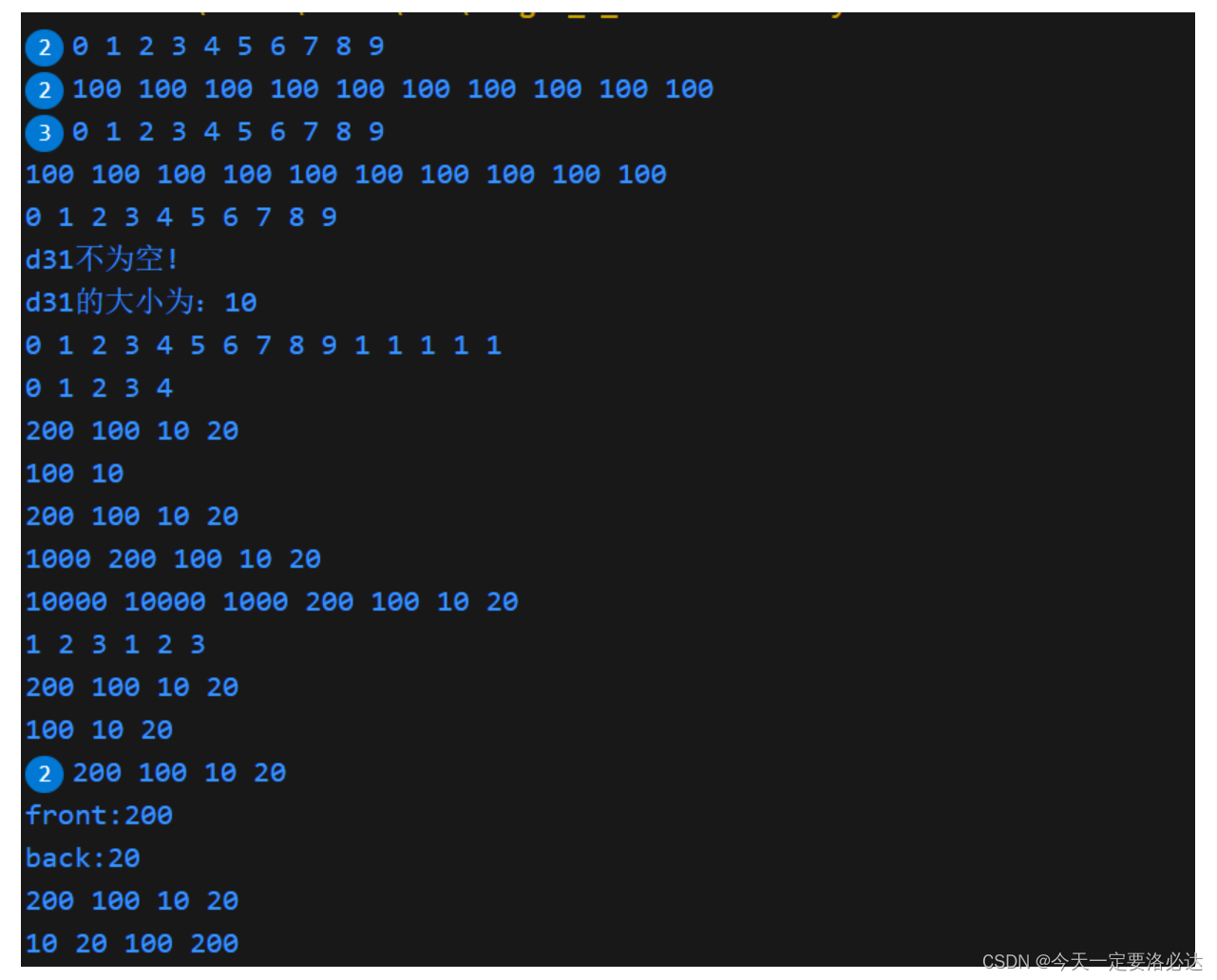## deque容器和Vector容器有何不同？

deque容器和vector容器是两种不同的容器类型，它们在内部实现和使用方式上有一些不同。

1. 内部实现：deque容器是双端数组（又一说为双端队列），它由多个连续的缓冲区组成，每个缓冲区可以存储多个元素。vector容器是动态数组，它在内存中以连续的方式存储元素。
2. 插入和删除操作：deque容器在两端进行插入和删除操作的时间复杂度都是O(1)，而vector容器在尾部进行插入和删除操作的时间复杂度也是O(1)，但在头部进行插入和删除操作的时间复杂度是O(n)，因为需要移动其他元素。
3. 访问元素：deque容器和vector容器都支持随机访问，即可以通过下标直接访问元素。但由于vector容器的元素在内存中是连续存储的，所以vector容器的随机访问速度更快。无论是deque容器还是vector容器，在已知元素的下标情况下，访问元素的时间复杂度都是O(1)这里说的随机访问是针对迭代器而言的
4. 内存分配：deque容器在内部使用多个缓冲区，每个缓冲区的大小可以根据需要动态调整。vector容器分配的内存空间是连续的，当容器大小增加时，可能需要重新分配更大的内存空间，并将原有元素复制到新的内存空间。

## 讲一讲deque容器的工作原理，缓冲区是什么，中控器又是什么？

deque（双端队列）是一种由多个连续的缓冲区组成的容器，它的内部工作原理涉及到缓冲区和中控器的概念。

## deque.begin()和deque.front(）效果一样吗

`deque.begin()`是一个成员函数，它返回一个迭代器，指向deque容器中的第一个元素。

`deque.front()`也是一个成员函数，它返回deque容器中的第一个元素的引用。

(其他容器也是相似的回答)

## string容器使用合集

``````#include<iostream>
#include<string>
#include<algorithm>
using namespace std;

int main(){
//string基础操作指令集(以int为例)
//1.构造方式4种
string s1="hello1";//无参构造方式
cout<<"s1="<<s1<<endl;

const char* str="hello2";
string s2(str);//将C语言风格字符串转换为C++风格字符串
cout<<"s2="<<s2<<endl;
string s3(s2);//调用拷贝构造函数 深拷贝
cout<<"s3="<<s3<<endl;

string s4(10,'a');//使用重载的构造函数
cout<<"s4="<<s4<<endl;

//2.赋值方式
string str1; //char*类型字符串 赋值给当前的字符串
str1 = "hello world";
cout << "str1 = " << str1 << endl;

string str2;
str2 = str1;//把字符串s赋给当前的字符串
cout << "str2 = " << str2 << endl;

string str3;//字符赋值给当前的字符串
str3 = 'a';
cout << "str3 = " << str3 << endl;

string str4; //把字符串s赋给当前字符串
str4.assign("hello c++");
cout << "str4 = " << str4 << endl;

string str5;
str5.assign("hello c++",5);//把字符串s的前n个字符赋给当前的字符串
cout << "str5 = " << str5 << endl;

string str6;
str6.assign(str5);//把字符串s赋给当前字符串
// 和str4的方法很相似 str4输入的参数是const char *s  str6输入的参数是const string &s
cout << "str6 = " << str6 << endl;

string str7;
str7.assign(5, 'x'); //用n个字符c赋给当前字符串
cout << "str7 = " << str7 << endl;
//3.字符串拼接
string str31 = "我";
str31 += "爱玩游戏";//重载+=操作符string& operator+=(const char* str);
cout << "str31 = " << str31 << endl;
str31 += ':';//重载+=操作符 string& operator+=(const char c);
cout << "str31 = " << str31 << endl;
string str32 = "LOL DNF";
str31 += str32;//重载+=操作符`string& operator+=(const string& str);`
cout << "str31 = " << str31 << endl;
string str33 = "I";
str33.append(" love ");//把字符串s连接到当前字符串结尾
str33.append("game abcde", 4);//把字符串s的前n个字符连接到当前字符串结尾
//str3.append(str2);
str33.append(str32, 4, 3); // 从下标4位置开始 ，截取3个字符，拼接到字符串末尾
cout << "str33 = " << str33 << endl;

//4.查找和替换
string str41 = "abcdefgde";
int pos = str41.find("de");//查找s第一次出现位置，找到了就返回下标（起始位置可以改）
if (pos == -1)
{
cout << "未找到" << endl;
}
else
{
cout << "pos = " << pos << endl;
}
pos = str41.rfind("de");//查找s最后一次出现位置
cout << "pos = " << pos << endl;
string str42 = "abcdefgde";
str42.replace(1, 3, "1111");//替换从pos开始的n个字符为字符串s
cout << "str1 = " << str42 << endl;
//5.字符串比较
string s51 = "hello";
string s52 = "aello";

int ret = s51.compare(s52);

if (ret == 0) {
cout << "s51 等于 s52" << endl;
}
else if (ret > 0)
{
cout << "s51 大于 s52" << endl;
}
else
{
cout << "s51 小于 s52" << endl;
}
//根据ASCII码表，h的ASCII码值大于a的ASCII码值。
//如果两个字符串的首字母相同，那么compare()函数会继续比较下一个字符，直到找到不同的字符或者其中一个字符串结束。
//6.字符存取
string str61 = "hello world";
//字符修改
str61 = 'x';//通过[]方式取字符
str61.at(1) = 'x';  //通过at方法获取字符
cout << str61 << endl;
//7.插入和删除
string str71 = "hello";
str71.insert(1, "111");//插入字符串
cout << str71 << endl;

str71.erase(1, 3);  //从1号位置开始3个字符,删除
cout << str71 << endl;
//8.子串
string str81 = "abcdefg";
string subStr81 = str81.substr(1, 3);//返回由pos开始的n个字符组成的字符串 pos是第一个参数
cout << "subStr81 = " << subStr81 << endl;

string email = "hello@sina.com";
int pos2 = email.find("@");
return 0;
}
``````## 介绍一下STL中stack queue 它们代表什么数据结构

std::stack是一个适配器容器，它使用其他容器作为底层数据结构，并提供了栈（LIFO）的功能。默认情况下，std::deque（双端队列）被用作std::stack的底层容器，但也可以使用std::vector或std::list等容器。

std::stack基于底层容器的特性，提供了高效的栈操作。它适用于需要栈功能的场景，如逆序输出、括号匹配、深度优先搜索等。

std::queue也是一个适配器容器，它使用其他容器作为底层数据结构，并提供了队列（FIFO）的功能。默认情况下，std::deque（双端队列）被用作std::queue的底层容器，但也可以使用std::list等容器。

std::queue基于底层容器的特性，提供了高效的队列操作。它适用于需要队列功能的场景，如广度优先搜索、任务调度等。

## 什么是适配器容器

``````std::stack<int, std::vector<int>> myStack;
``````

std::stack 的定义中，有两个模板参数，分别是 T 和 Container。

T 是栈中存储的元素类型。在你的例子中，T 被指定为 int，表示栈中存储的是整数类型的元素。

Container 是底层容器的类型。在你的例子中，Container 被指定为 std::vector，表示使用 std::vector 作为底层容器来实现栈的功能。

## stack容器使用合集

``````#include<iostream>
#include<stack>
#include<algorithm>
using namespace std;

int main(){
//list基础操作指令集(以int为例)
stack<int> s;

//向栈中添加元素，叫做 压栈 入栈
s.push(10);
s.push(20);
s.push(30);

while (!s.empty()) {
//输出栈顶元素
cout << "栈顶元素为： " << s.top() << endl;
//弹出栈顶元素
s.pop();
}
cout << "栈的大小为：" << s.size() << endl;

return 0;
}
``````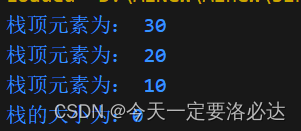## queue容器使用合集

``````#include<iostream>
#include<queue>
#include<algorithm>
using namespace std;
class Person
{
public:
Person(string name, int age)
{
this->m_Name = name;
this->m_Age = age;
}

string m_Name;
int m_Age;
};
int main(){
//queue基础操作指令集(以int为例)
//创建队列
queue<Person> q;

//准备数据
Person p1("唐僧", 30);
Person p2("孙悟空", 1000);
Person p3("猪八戒", 900);
Person p4("沙僧", 800);

//向队列中添加元素  入队操作
q.push(p1);
q.push(p2);
q.push(p3);
q.push(p4);

//队列不提供迭代器，更不支持随机访问
while (!q.empty()) {
//输出队头元素
cout << "队头元素-- 姓名： " << q.front().m_Name
<< " 年龄： "<< q.front().m_Age << endl;

cout << "队尾元素-- 姓名： " << q.back().m_Name
<< " 年龄： " << q.back().m_Age << endl;

cout << endl;
//弹出队头元素
q.pop();
}

cout << "队列大小为：" << q.size() << endl;

return 0;
}
``````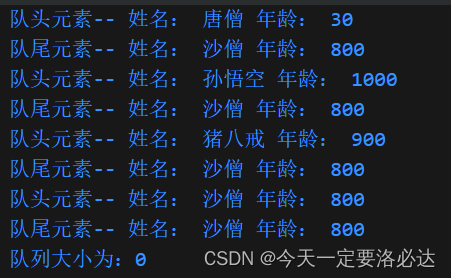## 介绍一下list容器，它是由哪种数据结构实现的？

list（链表）是C++标准库中的一种容器，它是一个双向链表，可以在任意位置进行高效的插入和删除操作。
list的特点是在插入和删除元素时具有良好的性能，无论是在容器的开头、结尾还是中间位置。这是因为链表的结构允许在常量时间内进行插入和删除操作，而不需要像数组那样重新分配和移动元素。（优点）

list还提供了一些特殊的操作，如在指定位置插入元素、合并两个有序的list、反转list等。这些操作在某些场景下非常有用，例如需要频繁插入和删除元素的情况。

## list容器使用合集

``````#include<iostream>
#include<list>
#include<algorithm>
using namespace std;

void printList(const list<int>& L) {

for (list<int>::const_iterator it = L.begin(); it != L.end(); it++) {
cout << *it << " ";
}
cout << endl;
}
bool myCompare(int val1 , int val2)
{
return val1 > val2;
}//自定义排序规则
int main(){
//list基础操作指令集(以int为例)
//1.构造方式
list<int>L1;
L1.push_back(10);
L1.push_back(20);
L1.push_back(30);
L1.push_back(40);

printList(L1);

list<int>L2(L1.begin(),L1.end());
printList(L2);

list<int>L3(L2);
printList(L3);

list<int>L4(10, 1000);
printList(L4);
//2.赋值和交换
list<int>L21;
L21.push_back(10);
L21.push_back(20);
L21.push_back(30);
L21.push_back(40);
printList(L21);

//赋值
list<int>L22;
L22 = L21;
printList(L22);

list<int>L23;
L23.assign(L22.begin(), L22.end());
printList(L23);

list<int>L24;
L24.assign(10, 100);
printList(L24);
//交换
list<int>L25;
L25.push_back(10);
L25.push_back(20);
L25.push_back(30);
L25.push_back(40);

list<int>L26;
L26.assign(10, 100);

cout << "交换前： " << endl;
printList(L25);
printList(L26);

cout << endl;

L25.swap(L26);

cout << "交换后： " << endl;
printList(L25);
printList(L26);
//3.大小操作
list<int>L31;
L31.push_back(10);
L31.push_back(20);
L31.push_back(30);
L31.push_back(40);

if (L31.empty())
{
cout << "L31为空" << endl;
}
else
{
cout << "L31不为空" << endl;
cout << "L31的大小为： " << L31.size() << endl;
}

//重新指定大小
L31.resize(10);
printList(L31);

L31.resize(2);
printList(L31);

//4.插入和删除
cout<<"插入和删除演示"<<endl;
list<int> L41;
//尾插
L41.push_back(10);
L41.push_back(20);
L41.push_back(30);
//头插
L41.push_front(100);
L41.push_front(200);
L41.push_front(300);

printList(L41);

//尾删
L41.pop_back();
printList(L41);

//头删
L41.pop_front();
printList(L41);

//插入
list<int>::iterator it = L41.begin();
L41.insert(++it, 1000);
printList(L41);

//删除
it = L41.begin();
L41.erase(++it);
printList(L41);

//移除
L41.push_back(10000);
L41.push_back(10000);
L41.push_back(10000);
printList(L41);
L41.remove(10000);
printList(L41);

//清空
L41.clear();
printList(L41);

//5.数据存取
list<int>L51;
L51.push_back(10);
L51.push_back(20);
L51.push_back(30);
L51.push_back(40);

//cout << L51.at(0) << endl;//错误 不支持at访问数据
//cout << L51 << endl; //错误  不支持[]方式访问数据
cout << "第一个元素为： " << L51.front() << endl;
cout << "最后一个元素为： " << L51.back() << endl;

//list容器的迭代器是双向迭代器，不支持随机访问
list<int>::iterator it11 = L51.begin();
//it = it + 1;//错误，不可以跳跃访问，即使是+1

//6.反转和排序
list<int> L6;
L6.push_back(90);
L6.push_back(30);
L6.push_back(20);
L6.push_back(70);
printList(L6);

//反转容器的元素
L6.reverse();
printList(L6);

//排序
L6.sort(); //默认的排序规则 从小到大
printList(L6);

L6.sort(myCompare); //指定规则，从大到小
//注意，这是类的成员函数sort，和算法库的sort不一样
printList(L6);

return 0;
}
``````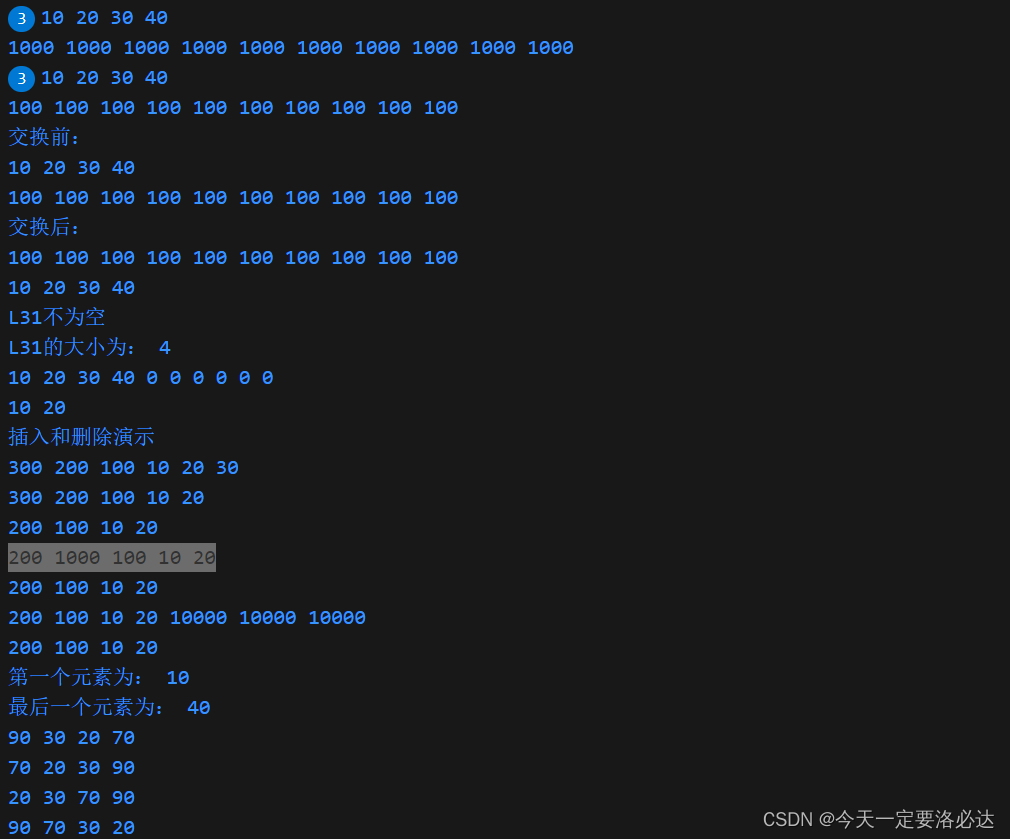## 讲一下vector deque string list stack queue各自常用功能的时间复杂度

`vector`:

• 时间复杂度：
• 随机访问：O(1)
• 插入和删除（在末尾以外的位置）：O(n)
• 插入和删除（在末尾位置）：平摊O(1)（近似O（1）吧）

`deque`:

• 时间复杂度：
• 随机访问：O(1)
• 插入和删除（在开头和末尾）：O(1)
• 插入和删除（在中间位置）：O(n)

`string`:

• 时间复杂度：
• 随机访问：O(1)
• 插入和删除（在末尾以外的位置）：O(n)
• 插入和删除（在末尾位置）：平摊O(1)

`list`:

• 时间复杂度：
• 随机访问：O(n)
• 插入和删除：O(1)

`stack`:

• 时间复杂度：
• 插入和删除（在栈顶）：O(1)
• 访问栈顶元素：O(1)

`queue`:

• 时间复杂度：
• 插入和删除（在队尾和队头）：O(1)
• 访问队头元素：O(1)

## STL的迭代器有几种

STL中的容器和算法通常会指定所需的迭代器类型，以确保它们能够正常工作。

## vector deque string list stack queue set map用的什么迭代器?

• vector：使用随机访问迭代器（Random Access Iterator）。由于vector支持常数时间的随机访问，因此使用随机访问迭代器可以高效地访问和修改元素。
• deque：使用随机访问迭代器（Random Access Iterator）。类似于vector，deque也支持常数时间的随机访问。
• string：使用随机访问迭代器（Random Access Iterator）。由于string本质上是字符序列，可以像访问数组一样使用随机访问迭代器来访问和修改字符。
• list：使用双向迭代器（Bidirectional Iterator）。由于list是双向链表，只支持双向遍历，因此使用双向迭代器可以遍历和修改list中的元素。
• stack：不支持迭代器。stack是一种后进先出（LIFO）的数据结构，只允许在栈顶进行插入和删除操作，无需遍历。
• queue：不支持迭代器。queue是一种先进先出（FIFO）的数据结构，只允许在队列的一端进行插入操作，在另一端进行删除操作，无需遍历。
• set：使用双向迭代器（Bidirectional Iterator）。set是一种有序、不重复的集合，使用双向迭代器可以遍历和修改set中的元素。
• map：使用双向迭代器（Bidirectional Iterator）。map是一种键值对的集合，使用双向迭代器可以遍历和修改map中的元素。

## 讲一下RAII（Resource Acquisition Is Initialization）（资源获取即初始化）

RAII（Resource Acquisition Is Initialization）是一种编程技术，用于在C++中管理资源的获取和释放。它是一种基于对象生命周期的管理方式，通过在对象的构造函数中获取资源，在对象的析构函数中释放资源，从而确保资源的正确释放。

1. 简化资源管理：通过将资源的获取和释放绑定到对象的生命周期，可以避免手动管理资源的复杂性。

2. 避免资源泄漏：由于资源的释放是在析构函数中进行的，即使在异常情况下，资源也能够得到正确的释放，避免资源泄漏。

3. 异常安全性：当使用RAII管理资源时，即使发生异常，对象的析构函数也会被调用，确保资源的正确释放，提供了更好的异常安全性。

4. 提高代码可读性和可维护性：RAII将资源的获取和释放逻辑封装在对象中，使得代码更加清晰、简洁和易于理解

1）智能指针是一种实现了RAII的重要工具。C++标准库中提供了几种智能指针类，如`std::unique_ptr``std::shared_ptr``std::weak_ptr`。这些智能指针类封装了指针，并在对象生命周期结束时自动释放指针所指向的资源。

2）基于作用域的资源管理：在C++中，可以利用作用域的特性来管理资源。通过在作用域内创建对象，在对象的析构函数中释放资源，可以实现简单的RAII。例如，使用局部对象、临时对象或函数内部类来管理资源。

3）RAII类：可以创建一个专门的RAII类来管理资源。这个类的构造函数负责获取资源，析构函数负责释放资源。通过使用这个RAII类的对象，可以确保资源在对象生命周期结束时被正确释放。

4）文件句柄封装：对于文件资源，可以创建一个封装文件句柄的类，类的构造函数打开文件，析构函数关闭文件。这样，在对象的生命周期结束时，文件句柄会被自动关闭。

5）锁的自动释放：在多线程编程中，可以使用互斥锁或信号量等同步原语来保护共享资源。通过在RAII类中封装锁的获取和释放操作，可以确保在对象生命周期结束时，锁会被自动释放，避免死锁和资源泄漏问题。

6）适当的资源管理库：有一些第三方库提供了更高级的资源管理功能，如Boost库中的`scoped_ptr``scoped_array`等。这些库提供了更多的RAII类和工具，以简化资源管理的操作。

## 介绍一下set容器，同时讲一下set和multiset的区别,

set是C++标准库中的一个容器，std::set的中文名叫集合。它提供了一种存储唯一元素的方式，即每个元素在set中都是唯一的，不会出现重复。
set容器内部使用红黑树（Red-Black Tree） 数据结构来实现元素的存储和排序。红黑树是一种自平衡二叉搜索树，具有良好的插入、删除和查找性能。
set容器的特点包括：

set和multiset都是C++标准库中提供的容器，**它们都是基于红黑树实现的，**并且都用于存储一组元素。它们之间的主要区别在于元素的唯一性和重复性。

set容器：set容器中的元素是唯一的，即每个元素只能出现一次。如果尝试插入一个已经存在的元素，插入操作将不会生效。set容器会自动根据元素的值进行排序，并且可以快速进行插入、删除和查找操作。

multiset容器：multiset容器中的元素允许重复即相同的元素可以出现多次。multiset容器会根据元素的值进行排序，并且可以快速进行插入、删除和查找操作。与set容器不同的是，multiset容器允许插入重复的元素，并且会按照排序规则保持这些重复元素的顺序。

## set和multiset，与unorderedset有何区别？

`std::set``std::multiset``std::unordered_set`这三个容器在实现方式和特性上有一些区别。

1. 有序性：`std::set``std::multiset`是有序的容器，元素按照其值进行排序。`std::set`只能存储唯一的元素，而`std::multiset`允许存储重复的元素。`std::unordered_set`是无序的容器，元素的顺序是不确定的。
2. 底层实现：`std::set``std::multiset`通常使用红黑树（Red-Black Tree）实现，这种数据结构保持了元素的有序性。`std::unordered_set`通常使用哈希表（Hash Table）（哈希映射
3. ）实现，这种数据结构通过哈希函数将元素映射到桶中，实现了快速的插入、删除和查找操作。
4. 时间复杂度：`std::set``std::multiset``std::unordered_set`在插入、删除和查找操作的平均时间复杂度上有所不同。`std::set``std::multiset`的平均时间复杂度是O(log n)，而`std::unordered_set`的平均时间复杂度是常数时间O(1)。然而，`std::unordered_set`在最坏情况下可能达到线性时间O(n)。（前两者之所以慢一点可能是因为树本身查找起来比较慢吧）
5. 元素顺序：`std::set``std::multiset`按照元素的值进行排序，所以元素的顺序是确定的。`std::unordered_set`不会对元素进行排序，所以元素的顺序是不确定的。

## set容器使用合集

``````#include<iostream>
#include<set>
#include<algorithm>
using namespace std;
//自定义数据类型
class Person
{
public:
Person(string name, int age)
{
this->m_Name = name;
this->m_Age = age;
}

string m_Name;
int m_Age;

};
//set自定义排序方法MyCompare(针对内置数据类型)
//下面用仿函数实现的
class MyCompare
{
public:
bool operator()(int v1, int v2) {
return v1 > v2;
}
};
//set自定义排序方法MyCompare(针对自定义数据类型)
//下面用仿函数实现的
class comparePerson
{
public:
bool operator()(const Person& p1, const Person &p2)
{
//按照年龄进行排序  降序
return p1.m_Age > p2.m_Age;
}
};
void printSet(set<int> & s)
{
for (set<int>::iterator it = s.begin(); it != s.end(); it++)
{
cout << *it << " ";
}
cout << endl;
}

int main(){

//set基础操作指令集(以int为例)
//1.构造方式
set<int> s1;
s1.insert(10);
s1.insert(30);
s1.insert(20);
s1.insert(40);
printSet(s1);
//拷贝构造
set<int>s2(s1);
printSet(s2);
//赋值
set<int>s3;
s3 = s2;
printSet(s3);

//2.求大小和交换
set<int> s21;

s21.insert(10);
s21.insert(30);
s21.insert(20);
s21.insert(40);

if (s21.empty())
{
cout << "s21为空" << endl;
}
else
{
cout << "s21不为空" << endl;
cout << "s21的大小为： " << s21.size() << endl;
}
//交换
set<int> s22;

s22.insert(10);
s22.insert(30);
s22.insert(20);
s22.insert(40);

set<int> s23;

s23.insert(100);
s23.insert(300);
s23.insert(200);
s23.insert(400);

cout << "交换前" << endl;
printSet(s22);
printSet(s23);
cout << endl;

cout << "交换后" << endl;
s22.swap(s23);
printSet(s22);
printSet(s23);

//3.插入和删除
set<int> s31;
//插入
s31.insert(10);
s31.insert(30);
s31.insert(20);
s31.insert(40);
printSet(s31);

//删除
s31.erase(s31.begin());
printSet(s31);

s31.erase(30);
printSet(s31);

//清空
//s1.erase(s1.begin(), s1.end());
s31.clear();
printSet(s31);
//4.查找和统计
set<int> s41;
//插入
s41.insert(10);
s41.insert(30);
s41.insert(20);
s41.insert(40);

//查找
set<int>::iterator pos = s41.find(30);

if (pos != s41.end())
{
cout << "找到了元素 ： " << *pos << endl;
}
else
{
cout << "未找到元素" << endl;
}

//统计
int num = s41.count(30);
cout << "num = " << num << endl;
//5.pair对组创建
pair<string, int> p(string("Tom"), 20);
cout << "姓名： " <<  p.first << " 年龄： " << p.second << endl;

pair<string, int> p2 = make_pair("Jerry", 10);
cout << "姓名： " << p2.first << " 年龄： " << p2.second << endl;
//6.排序
//6.1内置数据类型：
set<int> s61;
s61.insert(10);
s61.insert(40);
s61.insert(20);
s61.insert(30);
s61.insert(50);

//默认从小到大
for (set<int>::iterator it = s61.begin(); it != s61.end(); it++) {
cout << *it << " ";
}
cout << endl;

//指定排序规则
set<int,MyCompare> s62;
s62.insert(10);
s62.insert(40);
s62.insert(20);
s62.insert(30);
s62.insert(50);

for (set<int, MyCompare>::iterator it = s62.begin(); it != s62.end(); it++) {
cout << *it << " ";
}
cout << endl;
//6.2自定义类型：
set<Person, comparePerson> s63;

Person p1("刘备", 23);
Person p22("关羽", 27);
Person p3("张飞", 25);
Person p4("赵云", 21);

s63.insert(p1);
s63.insert(p22);
s63.insert(p3);
s63.insert(p4);

for (set<Person, comparePerson>::iterator it = s63.begin(); it != s63.end(); it++)
{
cout << "姓名： " << it->m_Name << " 年龄： " << it->m_Age << endl;
}

return 0;
}
``````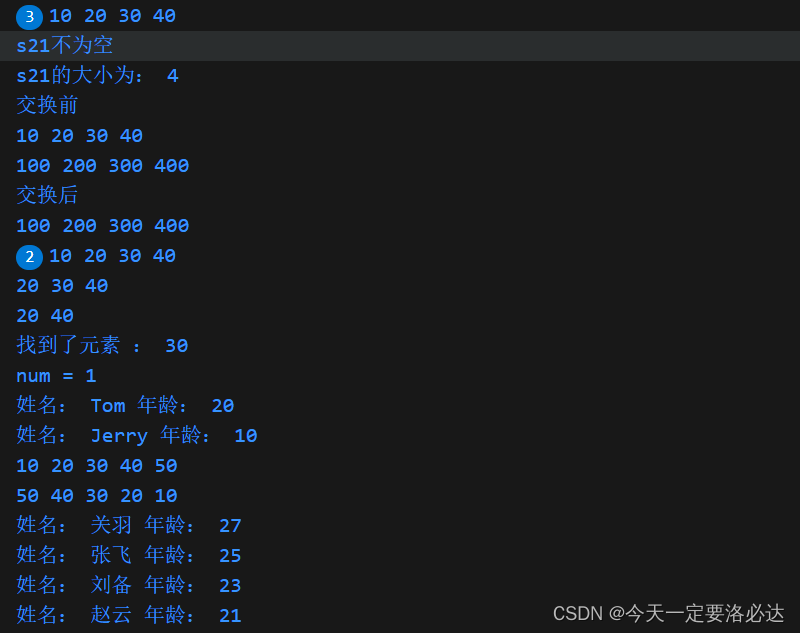## 讲一下set容器和map容器的区别

`set``map`是C++ STL中的两种不同的关联容器，它们在以下几个方面有所不同：

1. 存储方式：`set`容器存储唯一的键，而`map`容器存储键值对，其中每个键都是唯一的。
2. 排序和查找：`set`容器中的元素按照键的值进行排序，并且可以使用键进行快速查找。`map`容器中的元素按照键的值进行排序，并且可以通过键快速查找对应的值。
3. 插入和删除：在`set`容器中，可以使用`insert()`函数向容器中插入键。在`map`容器中，可以使用`insert()`函数或`[]`操作符来插入键值对。
4. 重复键：`set`容器中的键是唯一的，不允许重复。`map`容器中的键也是唯一的，不允许重复，但是可以使用键来更新对应的值。
``````#include <iostream>
#include <set>
#include <map>

int main() {
// set容器示例
std::set<int> mySet;
mySet.insert(1);
mySet.insert(2);
mySet.insert(3);

for (const auto& element : mySet) {
std::cout << "Set Key: " << element << std::endl;
}

// map容器示例
std::map<int, std::string> myMap;
myMap.insert({1, "Apple"});
myMap.insert({2, "Banana"});
myMap.insert({3, "Orange"});

for (const auto& pair : myMap) {
std::cout << "Map Key: " << pair.first << ", Value: " << pair.second << std::endl;
}

return 0;
}
``````

## 为什么set容器里要考虑pair对组这个概念？

``````#include <iostream>
#include <set>

int main() {
std::set<std::pair<int, std::string>> mySet;

mySet.insert(std::make_pair(1, "Apple"));
mySet.insert(std::make_pair(2, "Banana"));
mySet.insert(std::make_pair(3, "Orange"));

for (const auto& pair : mySet) {
std::cout << "Key: " << pair.first << ", Value: " << pair.second << std::endl;
}

return 0;
}
``````

``````#include <iostream>
#include <set>

int main() {
std::set<std::pair<int, std::string>> mySet;

mySet.insert(std::make_pair(1, "Apple"));
mySet.insert(std::make_pair(2, "Banana"));
mySet.insert(std::make_pair(1, "Orange"));  // 与第一个键值对的键值相同，不会被插入

for (const auto& pair : mySet) {
std::cout << "Key: " << pair.first << ", Value: " << pair.second << std::endl;
}

return 0;
}
``````

Key: 1, Value: Apple
Key: 2, Value: Banana

1）当我们遍历一个容器时，如果我们只是想读取容器中的元素而不修改它们，最好使用const修饰循环变量。这样可以确保我们不会意外地修改容器中的元素。
2）使用const auto& pair则通过引用的方式访问容器中的元素，避免了拷贝操作，提高了效率。

## set<>尖括号里可以放几个参数

``````std::set<int, std::greater<int>> s; // 这会创建一个按照降序排列的 set
``````

## 讲一下map和mutimap的区别

map的中文名叫"映射"

1. 键的唯一性：在`std::map`中，每个键只能对应一个值。如果尝试插入一个已经存在的键，它将不会插入新的值，而是更新现有键对应的值。这使得`std::map`适用于需要维护唯一键值对的情况。
2. 键的重复性：与之相反，在`std::multimap`中，允许相同的键对应多个值。这意味着你可以插入具有相同键的多个值，并且每个值都会被保留。这使得`std::multimap`适用于需要存储键值对且允许键重复的情况。
``````#include <iostream>
#include <map>
#include <string>

int main() {
std::multimap<int, std::string> myMultiMap;
myMultiMap.insert(std::make_pair(1, "Apple"));
myMultiMap.insert(std::make_pair(2, "Banana"));
myMultiMap.insert(std::make_pair(1, "Orange")); // 相同的键，不同的值
myMultiMap.insert(std::make_pair(3, "Apple"));

for (const auto& pair : myMultiMap) {
std::cout << "Key: " << pair.first << ", Value: " << pair.second << std::endl;
}

return 0;
}
``````

Key: 1, Value: Apple
Key: 1, Value: Orange
Key: 2, Value: Banana
Key: 3, Value: Apple

1. 元素排序：`std::map``std::multimap`都可以提供有序性。`std::map`使用红黑树（Red-Black Tree）实现，它根据键的顺序进行排序。而`std::multimap`也使用红黑树实现，但它允许相同的键存在，因此它在排序时不会合并相同的键。

## 如何判断map中哪个是键哪个是值；如何访问键和值？

``````#include <iostream>
#include <map>
#include <string>

int main() {
std::map<int, std::string> myMap;
myMap.insert(std::make_pair(1, "Apple"));
myMap.insert(std::make_pair(2, "Banana"));
myMap.insert(std::make_pair(3, "Orange"));

for (const auto& pair : myMap) {
std::cout << "Key: " << pair.first << ", Value: " << pair.second << std::endl;
}

return 0;
}

``````

Key: 1, Value: Apple
Key: 2, Value: Banana
Key: 3, Value: Orange

## map，mutimap和unordered_map的区别

`std::map``std::multimap``std::unordered_map`是C++标准库中的三种不同的关联容器，它们具有以下区别：

1. 有序性：
• `std::map`是一个有序关联容器，根据键进行排序，默认情况下按照升序排列。
• `std::multimap`也是一个有序关联容器，允许插入具有相同键的多个元素，并根据键进行排序。
• `std::unordered_map`是一个无序关联容器，元素的顺序不依赖于键的值，而是由哈希函数决定
2. 底层实现：
• `std::map``std::multimap`通常使用红黑树实现，这使得它们在插入、删除和查找元素时具有较好的性能。
• `std::unordered_map`使用哈希表实现，这使得它在平均情况下具有常数时间的插入、删除和查找操作。
3. 键的唯一性：
• `std::map`要求键是唯一的，如果插入具有相同键的元素，旧的元素将被新的元素替换。
• `std::multimap`允许插入具有相同键的多个元素。
• `std::unordered_map`要求键是唯一的，如果插入具有相同键的元素，旧的元素将被新的元素替换。
4. 性能特点：
• `std::map``std::multimap`适用于需要有序访问元素的场景，它们提供了一些有关元素顺序的功能。
• `std::unordered_map`适用于需要快速查找元素的场景，它的插入、删除和查找操作具有较好的平均性能。

## map容器使用合集

``````#include<iostream>
#include<map>
#include<algorithm>
using namespace std;

void printMap(map<int,int>&m)
{
for (map<int, int>::iterator it = m.begin(); it != m.end(); it++)
{
cout << "key = " << it->first << " value = " << it->second << endl;
}
cout << endl;
}
//自定义排序的仿函数
class MyCompare {
public:
bool operator()(int v1, int v2) {
return v1 > v2;
}
};
int main(){
//map基础操作指令集(以int为例)
//1.构造方式
map<int,int>m; //默认构造
m.insert(pair<int, int>(1, 10));
m.insert(pair<int, int>(2, 20));
m.insert(pair<int, int>(3, 30));
printMap(m);

map<int, int>m2(m); //拷贝构造
printMap(m2);

map<int, int>m3;
m3 = m2; //赋值
printMap(m3);

//2.大小和交换
map<int, int>m21;
m21.insert(pair<int, int>(1, 10));
m21.insert(pair<int, int>(2, 20));
m21.insert(pair<int, int>(3, 30));

if (m21.empty())
{
cout << "m21为空" << endl;
}
else
{
cout << "m21不为空" << endl;
cout << "m21的大小为： " << m21.size() << endl;
}

//交换
map<int, int>m22;
m22.insert(pair<int, int>(1, 10));
m22.insert(pair<int, int>(2, 20));
m22.insert(pair<int, int>(3, 30));

map<int, int>m23;
m23.insert(pair<int, int>(4, 100));
m23.insert(pair<int, int>(5, 200));
m23.insert(pair<int, int>(6, 300));

cout << "交换前" << endl;
printMap(m22);
printMap(m23);

cout << "交换后" << endl;
m22.swap(m23);
printMap(m22);
printMap(m23);
cout<<"插入删除展示："<<endl;
//3.插入和删除
//插入
map<int, int> m31;
//第一种插入方式
m31.insert(pair<int, int>(1, 10));
//第二种插入方式
m31.insert(make_pair(2, 20));
//第三种插入方式
m31.insert(map<int, int>::value_type(3, 30));
//第四种插入方式
m31 = 40;
printMap(m31);

//删除
m31.erase(m31.begin());
printMap(m31);

m31.erase(3);
printMap(m31);

//清空
m31.erase(m31.begin(),m31.end());
m31.clear();
printMap(m31);
//4.查找和统计
map<int, int>m41;
m41.insert(pair<int, int>(1, 10));
m41.insert(pair<int, int>(2, 20));
m41.insert(pair<int, int>(3, 30));

//查找
map<int, int>::iterator pos = m41.find(3);

if (pos != m41.end())
{
cout << "找到了元素 key = " << (*pos).first << " value = " << (*pos).second << endl;
}// 这里用pos->first是等价的
else
{
cout << "未找到元素" << endl;
}

//统计
int num = m41.count(3);
cout << "num = " << num << endl;

//5.排序
map<int, int, MyCompare> m51;

m51.insert(make_pair(1, 10));
m51.insert(make_pair(2, 20));
m51.insert(make_pair(3, 30));
m51.insert(make_pair(4, 40));
m51.insert(make_pair(5, 50));

for (map<int, int, MyCompare>::iterator it = m51.begin(); it != m51.end(); it++) {
cout << "key:" << it->first << " value:" << it->second << endl;
}
return 0;
}
``````

## 讲一些STL中的back_inserter

std::back_inserter() 是一个函数模板，用于在容器的末尾插入元素的迭代器。它接受一个容器作为参数，并返回一个插入器迭代器，可以通过该迭代器将元素插入到容器的末尾。

``````#include <iostream>
#include <vector>
#include <iterator>
#include <algorithm>

int main() {
std::vector<int> nums = {1, 2, 3};

std::vector<int> destination;

std::copy(nums.begin(), nums.end(), std::back_inserter(destination));

for (const auto& num : destination) {
std::cout << num << " ";
}

return 0;
}
``````

std::copy() 函数接受三个参数：源容器的起始迭代器 nums.begin()、源容器的结束迭代器 nums.end()，以及目标容器的插入器迭代器 std::back_inserter(destination)

## 讲一些STL中你常用的算法

std::sort()：用于对容器中的元素进行排序，默认情况下按升序排序。可以自定义比较函数来实现不同的排序方式。

``````#include <iostream>
#include <vector>
#include <algorithm>

int main() {
std::vector<int> nums = {5, 2, 8, 1, 9};

std::sort(nums.begin(), nums.end());

for (const auto& num : nums) {
std::cout << num << " ";
}

return 0;
}
``````

std::find()：在容器中查找指定值的元素，并返回该元素的迭代器。如果找不到，则返回容器的 end() 迭代器。

``````#include <iostream>
#include <vector>
#include <algorithm>

int main() {
std::vector<int> nums = {5, 2, 8, 1, 9};

auto it = std::find(nums.begin(), nums.end(), 8);

if (it != nums.end()) {
std::cout << "Element found at index: " << std::distance(nums.begin(), it) << std::endl;
//std::distance() 是一个用于计算两个迭代器之间距离的函数
} else {
}

return 0;
}
``````

std::copy()：将一个容器的元素复制到另一个容器中，可以指定目标容器的起始位置。

``````#include <iostream>
#include <vector>
#include <algorithm>

int main() {
std::vector<int> source = {1, 2, 3, 4, 5};
std::vector<int> destination;

std::copy(source.begin(), source.end(), std::back_inserter(destination));

for (const auto& num : destination) {
std::cout << num << " ";
}

return 0;
}
``````

std::transform()：对一个容器的元素进行转换，并将结果存储到另一个容器中，可以指定转换函数。

``````#include <iostream>
#include <vector>
#include <algorithm>

int main() {
std::vector<int> nums = {1, 2, 3, 4, 5};
std::vector<int> squares;

std::transform(nums.begin(), nums.end(), std::back_inserter(squares), [](int num) {
return num * num;
});

for (const auto& square : squares) {
std::cout << square << " ";
}

return 0;
}

``````

std::accumulate()：对容器中的元素进行累加、求和等操作，可以指定起始值和操作函数。

``````#include <iostream>
#include <vector>
#include <numeric>

int main() {
std::vector<int> nums = {1, 2, 3, 4, 5};

int sum = std::accumulate(nums.begin(), nums.end(), 0);

std::cout << "Sum: " << sum << std::endl;

return 0;
}
``````

std::count()：统计容器中等于指定值的元素的个数。

``````#include <iostream>
#include <vector>
#include <algorithm>

int main() {
std::vector<int> nums = {1, 2, 2, 3, 2, 4, 2};

int count = std::count(nums.begin(), nums.end(), 2);

std::cout << "Count: " << count << std::endl;

return 0;
}
``````

std::replace()：将容器中等于指定值的元素替换为新值。

``````#include <iostream>
#include <vector>
#include <algorithm>

int main() {
std::vector<int> nums = {1, 2, 3, 4, 5};

std::replace(nums.begin(), nums.end(), 3, 10);

for (const auto& num : nums) {
std::cout << num << " ";
}

return 0;
}
``````

std::unique()：移除容器中的连续重复元素，只保留一个。

``````#include <iostream>
#include <vector>
#include <algorithm>

int main() {
std::vector<int> nums = {1, 2, 2, 3, 3, 3, 4, 4, 4, 4};

auto it = std::unique(nums.begin(), nums.end());
nums.erase(it, nums.end());

for (const auto& num : nums) {
std::cout << num << " ";
}

return 0;
}
``````

std::for_each()：对容器中的每个元素执行指定的操作，可以是函数、函数对象或lambda表达式。

``````#include <iostream>
#include <vector>
#include <algorithm>

void printSquare(int num) {
std::cout << num * num << " ";
}

int main() {
std::vector<int> nums = {1, 2, 3, 4, 5};

std::for_each(nums.begin(), nums.end(), printSquare);

return 0;
}

``````

std::binary_search()：在有序容器中进行二分查找，判断指定值是否存在。

``````#include <iostream>
#include <vector>
#include <algorithm>

int main() {
std::vector<int> nums = {1, 2, 3, 4, 5};

bool found = std::binary_search(nums.begin(), nums.end(), 3);

if (found) {
std::cout << "Element found" << std::endl;
} else {
}

return 0;
}
``````

## 成员函数push_back emplace_back的区别

push_back() 和 emplace_back() 是用于向容器的末尾添加元素的两个函数。

push_back()：这是一个成员函数，用于将一个对象添加到容器的末尾。它接受一个参数，即要添加的元素的值或引用。如果容器是动态数组（如 std::vector），push_back() 会在容器的末尾分配新的内存，并将元素复制或移动到新的位置。

``````std::vector<int> myVector;
myVector.push_back(10); // 添加元素 10 到 myVector 的末尾
``````

emplace_back()：这也是一个成员函数，用于在容器的末尾就地构造一个新的对象。它接受多个参数，这些参数将用于构造新对象的构造函数。与 push_back() 不同，emplace_back() 不需要复制或移动元素，而是直接在容器的内存中构造新对象。

``````std::vector<std::string> myVector;
myVector.emplace_back("Hello"); // 在 myVector 的末尾构造一个新的 std::string 对象
``````

emplace_back() 的优势在于避免了不必要的对象复制或移动操作，因为它直接在容器的内存中构造新对象。这对于构造开销较大的对象（如自定义类）尤其有用。但请注意，由于 emplace_back() 是就地构造对象，因此参数必须与容器存储的对象类型的构造函数相匹配。

## 讲一些STL中功能相似但不同的成员函数

push_back() vs insert():

push_back() 用于在容器的末尾插入一个元素。

insert() 可以在容器的任意位置插入一个或多个元素。

pop_back() vs erase():

pop_back() 用于删除容器的最后一个元素。

erase() 可以删除容器中的一个或多个元素，可以指定要删除的位置或者使用迭代器指定要删除的范围。

front() vs begin():

front() 用于访问容器的第一个元素。

begin() 返回一个指向容器中第一个元素的迭代器。

back() vs end():

back() 用于访问容器的最后一个元素。

end() 返回一个指向容器末尾（即最后一个元素之后）的迭代器。

size() vs empty():

size() 返回容器中元素的数量。

empty() 检查容器是否为空，返回一个布尔值。

## 讲一下 for_each transform两种遍历算法的区别

for_each和transform是STL中两个不同的遍历算法，它们的区别在于它们的目的和使用方式。

for_each：

``````#include <iostream>
#include <vector>
#include <algorithm>

void printElement(int value) {
std::cout << value << " ";
}

int main() {
std::vector<int> numbers = {1, 2, 3, 4, 5};
std::for_each(numbers.begin(), numbers.end(), printElement); // 输出：1 2 3 4 5
return 0;
}
``````

transform：

``````#include <iostream>
#include <vector>
#include <algorithm>

int square(int value) {
return value * value;
}

int main() {
std::vector<int> numbers = {1, 2, 3, 4, 5};
std::vector<int> squaredNumbers;

std::transform(numbers.begin(), numbers.end(), std::back_inserter(squaredNumbers), square);

// 输出原始容器和新容器的内容
for (int num : numbers) {
std::cout << num << " "; // 输出：1 2 3 4 5
}

std::cout << std::endl;

for (int num : squaredNumbers) {
std::cout << num << " "; // 输出：1 4 9 16 25
}

return 0;
}
``````

## 讲一下 find find_if adjacent_find binary_search count count_if等查找算法的区别

find, find_if, adjacent_find, binary_search, count, 和 count_if 是STL中常见的查找算法，它们用于在容器中查找元素或计算满足特定条件的元素个数。下面解释它们的区别：

find：

``````#include <iostream>
#include <vector>
#include <algorithm>

int main() {
std::vector<int> numbers = {1, 2, 3, 4, 5};

auto it = std::find(numbers.begin(), numbers.end(), 3);

if (it != numbers.end()) {
std::cout << "Found: " << *it << std::endl; // 输出：Found: 3
} else {
}

return 0;
}
``````

find_if：

``````#include <iostream>
#include <vector>
#include <algorithm>

bool isEven(int num) {
return num % 2 == 0;
}

int main() {
std::vector<int> numbers = {1, 2, 3, 4, 5};

auto it = std::find_if(numbers.begin(), numbers.end(), isEven);

if (it != numbers.end()) {
std::cout << "Found: " << *it << std::endl; // 输出：Found: 2
} else {
}

return 0;
}
``````

``````#include <iostream>
#include <vector>
#include <algorithm>

int main() {
std::vector<int> numbers = {1, 2, 2, 3, 4};

if (it != numbers.end()) {
std::cout << "Found: " << *it << std::endl; // 输出：Found: 2
} else {
}

return 0;
}
``````

binary_search：

``````#include <iostream>
#include <vector>
#include <algorithm>

int main() {
std::vector<int> numbers = {1, 2, 3, 4, 5};

bool found = std::binary_search(numbers.begin(), numbers.end(), 3);

if (found) {
std::cout << "Found" << std::endl;
} else {
}

return 0;
}
``````

count：

``````#include <iostream>
#include <vector>
#include <algorithm>

int main() {
std::vector<int> numbers = {1, 2, 2, 3, 2, 4};

int numTwos = std::count(numbers.begin(), numbers.end(), 2);

std::cout << "Number of twos: " << numTwos << std::endl; // 输出：Number of twos: 3

return 0;
}
``````

count_if：

``````#include <iostream>
#include <vector>
#include <algorithm>

bool isEven(int num) {
return num % 2 == 0;
}

int main() {
std::vector<int> numbers = {1, 2, 3, 4, 5};

int evenCount = std::count_if(numbers.begin(), numbers.end(), isEven);

std::cout << "Number of even elements: " << evenCount << std::endl; // 输出：Number of even elements: 2

return 0;
}
``````

## 讲一下 sort random_shuffle merge reverse等排序算法的区别

sort，random_shuffle，merge，和reverse是STL中常见的排序和重排算法，它们用于对容器中的元素进行排序和重新排列。下面解释它们的区别：

sort：

``````#include <iostream>
#include <vector>
#include <algorithm>

int main() {
std::vector<int> numbers = {5, 2, 1, 4, 3};

std::sort(numbers.begin(), numbers.end());

// 输出排序后的结果：1 2 3 4 5
for (int num : numbers) {
std::cout << num << " ";
}

return 0;
}
``````

random_shuffle：

``````#include <iostream>
#include <vector>
#include <algorithm>

int main() {
std::vector<int> numbers = {1, 2, 3, 4, 5};

std::random_shuffle(numbers.begin(), numbers.end());

// 输出随机排列后的结果，例如可能是：3 2 1 5 4
for (int num : numbers) {
std::cout << num << " ";
}

return 0;
}
``````

merge：

``````#include <iostream>
#include <vector>
#include <algorithm>

int main() {
std::vector<int> numbers1 = {1, 3, 5};
std::vector<int> numbers2 = {2, 4, 6};
std::vector<int> mergedNumbers(6);

std::merge(numbers1.begin(), numbers1.end(), numbers2.begin(), numbers2.end(), mergedNumbers.begin());

// 输出合并后的结果：1 2 3 4 5 6
for (int num : mergedNumbers) {
std::cout << num << " ";
}

return 0;
}
``````

reverse：

``````#include <iostream>
#include <vector>
#include <algorithm>

int main() {
std::vector<int> numbers = {1, 2, 3, 4, 5};

std::reverse(numbers.begin(), numbers.end());

// 输出逆序后的结果：5 4 3 2 1
for (int num : numbers) {
std::cout << num << " ";
}

return 0;
}
``````

## 讲一下 copy,replace,replace_if,swap等拷贝替换算法的区别

copy，replace，replace_if，和swap 是STL中常见的拷贝和替换算法，它们用于在容器中进行元素的拷贝和替换操作。下面解释它们的区别：

copy：

``````#include <iostream>
#include <vector>
#include <algorithm>

int main() {
std::vector<int> source = {1, 2, 3, 4, 5};
std::vector<int> destination(5);

std::copy(source.begin(), source.end(), destination.begin());

// 输出目标容器的内容：1 2 3 4 5
for (int num : destination) {
std::cout << num << " ";
}

return 0;
}
``````

replace：

``````#include <iostream>
#include <vector>
#include <algorithm>

int main() {
std::vector<int> numbers = {1, 2, 2, 3, 4, 2};

std::replace(numbers.begin(), numbers.end(), 2, 99);

// 输出替换后的结果：1 99 99 3 4 99
for (int num : numbers) {
std::cout << num << " ";
}

return 0;
}
``````

replace_if：

``````#include <iostream>
#include <vector>
#include <algorithm>

bool isEven(int num) {
return num % 2 == 0;
}

int main() {
std::vector<int> numbers = {1, 2, 3, 4, 5};

std::replace_if(numbers.begin(), numbers.end(), isEven, 0);

// 输出替换偶数后的结果：1 0 3 0 5
for (int num : numbers) {
std::cout << num << " ";
}

return 0;
}
``````

swap：

``````#include <iostream>
#include <vector>
#include <algorithm>

int main() {
int a = 10;
int b = 20;

std::swap(a, b);

std::cout << "a: " << a << ", b: " << b << std::endl; // 输出：a: 20, b: 10

return 0;
}
``````

## 讲一下accumulate，fill等算术生成算法的区别

accumulate 和 fill 是STL中的算术生成算法，它们用于在容器中进行数值计算和填充操作。下面解释它们的区别：

accumulate：

``````#include <iostream>
#include <vector>
#include <numeric> // 包含accumulate算法

int main() {
std::vector<int> numbers = {1, 2, 3, 4, 5};

int sum = std::accumulate(numbers.begin(), numbers.end(), 0);

std::cout << "Sum: " << sum << std::endl; // 输出：Sum: 15

return 0;
}
``````

``````#include <iostream>
#include <vector>
#include <numeric> // 包含accumulate算法

int main() {
std::vector<int> numbers = {1, 2, 3, 4, 5};

int product = std::accumulate(numbers.begin(), numbers.end(), 1, std::multiplies<int>());

std::cout << "Product: " << product << std::endl; // 输出：Product: 120

return 0;
}
``````

fill：

``````#include <iostream>
#include <vector>
#include <algorithm>

int main() {
std::vector<int> numbers(5);

std::fill(numbers.begin(), numbers.end(), 42);

// 输出填充后的结果：42 42 42 42 42
for (int num : numbers) {
std::cout << num << " ";
}

return 0;
}
``````

## 讲一下 set_intersection,set_union,set_difference等集合算法的区别

set_intersection，set_union，和 set_difference 是STL中的集合算法，它们用于操作有序容器（通常是已排序的）来进行交集、并集和差集操作。下面解释它们的区别：

set_intersection：

``````#include <iostream>
#include <vector>
#include <algorithm>

int main() {
std::vector<int> set1 = {1, 2, 3, 4, 5};
std::vector<int> set2 = {3, 4, 5, 6, 7};
std::vector<int> intersection(5);

std::set_intersection(set1.begin(), set1.end(), set2.begin(), set2.end(), intersection.begin());

// 输出交集的结果：3 4 5
for (int num : intersection) {
std::cout << num << " ";
}

return 0;
}
``````

set_union：

``````#include <iostream>
#include <vector>
#include <algorithm>

int main() {
std::vector<int> set1 = {1, 2, 3, 4, 5};
std::vector<int> set2 = {3, 4, 5, 6, 7};
std::vector<int> unionSet(10);

std::set_union(set1.begin(), set1.end(), set2.begin(), set2.end(), unionSet.begin());

// 输出并集的结果：1 2 3 4 5 6 7
for (int num : unionSet) {
std::cout << num << " ";
}

return 0;
}
``````

set_difference：

``````#include <iostream>
#include <vector>
#include <algorithm>

int main() {
std::vector<int> set1 = {1, 2, 3, 4, 5};
std::vector<int> set2 = {3, 4, 5, 6, 7};
std::vector<int> difference(5);

std::set_difference(set1.begin(), set1.end(), set2.begin(), set2.end(), difference.begin());

// 输出差集的结果：1 2
for (int num : difference) {
std::cout << num << " ";
}

return 0;
}
``````

## 讲一下STL的空间配置器

STL（标准模板库）的空间配置器是用于分配和管理内存的组件它负责在需要时分配内存，并在不再需要时释放内存，以支持容器（如vector、list、map等）和其他STL组件的动态存储需求。
STL的空间配置器使用了一种称为"allocator"的设计模式。它通过提供allocate()和deallocate()等函数来分配和释放内存，使得容器可以根据需要动态地分配和释放内存空间。
STL的空间配置器通常使用底层的内存分配函数（如malloc()和free()）来执行实际的内存分配和释放操作。它还可以管理内存池，以提高内存分配的效率。

## 以Vector为例，讲一下STL的空间配置器如何工作的

1）内存分配：当vector需要分配内存来存储元素时，它会调用空间配置器的allocate函数来请求一块足够大的内存空间。空间配置器会根据需要的内存大小，使用底层的内存分配函数（如malloc()或new）向操作系统申请内存。

2）内存管理：空间配置器会将所分配的内存空间进行管理，通常会记录已分配和未分配的内存块的状态。这样，当vector需要增加或减少元素时，空间配置器可以快速定位到可用的内存块。

3）内存释放：当vector不再需要某个内存块时，它会调用空间配置器的deallocate函数将该内存块返回给空间配置器。空间配置器会将该内存块标记为可用状态，以便下次分配时可以重新使用。

## 讲一下空间配置器的两层结构

1）第一级配置器是一个简单的分配器，它负责处理较大的内存分配请求。它使用的是全局的::operator new()和::operator delete()函数来执行内存的分配和释放。这些全局函数通常是直接调用底层的内存分配函数（如malloc()和free()）来完成实际的内存操作。
2）第二级配置器是一个更复杂的分配器，它负责处理较小的内存分配请求。它使用了一个内存池（memory pool），即一个已经预先分配好的大块内存区域。当需要分配内存时，第二级配置器会从内存池中获取一块足够大小的内存块，并将其分配给请求者。如果内存池中的内存块不足，第二级配置器会调用第一级配置器来执行更大的内存分配。

## 讲一下内存池

1）预分配内存：在程序启动时，内存池会向操作系统请求一块较大的内存区域。这块内存区域通常是连续的，并且足够大以满足程序的内存需求。

2）分配内存：当程序需要分配内存时，内存池会从预分配的内存区域中获取一块足够大小的内存块，并将其分配给程序。这个过程通常比直接向操作系统请求内存更快，因为内存池无需频繁地与操作系统进行通信。

3）释放内存：当程序不再需要某个内存块时，它可以将该内存块返回给内存池，而不是直接释放给操作系统。内存池会将这个内存块标记为可用状态，以便下次分配时可以重新使用。

1）减少内存碎片：由于内存池使用连续的内存区域，它可以减少内存碎片的产生。这是因为内存池中的内存块是紧密排列的，不会出现碎片化的情况。

2）提高内存分配效率：内存池避免了频繁的内存分配操作，通过预先分配一块较大的内存区域，可以减少内存分配的开销，提高内存分配的效率。

3）控制内存使用：内存池可以限制程序使用的内存总量，避免内存泄漏和过度分配的问题。通过预分配内存池的大小，可以控制程序的内存使用情况。

## 讲一下仿函数

``````class AddFunctor
{
public:
int operator()(int a, int b)
{
return a + b;
}
};

int main()
{
int result = add(3, 4); // 调用仿函数
// result = 7
return 0;
}
``````

## 一元谓词和二元谓词的区别是什么？

``````struct IsEven
{
bool operator()(int num)
{
return num % 2 == 0;
}
};

int main()
{
IsEven isEven;
bool result = isEven(4); // 调用一元谓词
// result = true
return 0;
}
``````

``````struct CompareLength
{
bool operator()(const std::string& str1, const std::string& str2)
{
return str1.length() < str2.length();
}
};

int main()
{
CompareLength compare;
bool result = compare("apple", "banana"); // 调用二元谓词
// result = true
return 0;
}
``````

## 介绍一下算术仿函数 关系仿函数 逻辑仿函数

``````#include <iostream>
#include <functional>

int main() {
std::plus<int> plus_obj;
std::minus<int> minus_obj;
std::cout << "10 + 5 = " << plus_obj(10, 5) << std::endl;
std::cout << "10 - 5 = " << minus_obj(10, 5) << std::endl;
return 0;
}
``````

10 + 5 = 15
10 - 5 = 5

``````#include <iostream>
#include <functional>

int main() {
std::less<int> less_obj;
std::greater<int> greater_obj;
std::cout << "Is 10 < 5 ? " << (less_obj(10, 5) ? "true" : "false") << std::endl;
std::cout << "Is 10 > 5 ? " << (greater_obj(10, 5) ? "true" : "false") << std::endl;
return 0;
}
``````

Is 10 < 5 ? false
Is 10 > 5 ? true

``````#include <iostream>
#include <functional>

int main() {
std::logical_and<bool> and_obj;
std::logical_or<bool> or_obj;
std::cout << "true AND false = " << (and_obj(true, false) ? "true" : "false") << std::endl;
std::cout << "true OR false = " << (or_obj(true, false) ? "true" : "false") << std::endl;
return 0;
}
``````

true AND false = false
true OR false = true

### JAVA面试总结-Redis篇章(六)——数据过期策略

Java面试总结-Redis篇章&#xff08;六&#xff09;——数据过期策略 Redis数据删除策略——惰性删除Redis数据删除策略——定期删除 Redis数据删除策略——惰性删除 Redis数据删除策略——定期删除

ADS仿真低噪声放大器 文章目录 ADS仿真低噪声放大器1. 安装晶体管的库文件2. 直流分析DC Tracing3. 偏置电路的设计4. 稳定性分析5. 输入匹配和输出匹配 设计要求&#xff1a; 工作频率&#xff1a;2.4~2.5GHz ISM频段 噪声系数&#xff1a;NF < 0.7 增益&#xff1a;Gain &…

### 13.5 【Linux】使用者的特殊 shell 与 PAM 模块

13.5.1 特殊的 shell, /sbin/nologin 我们所谓的“无法登陆”指的仅是&#xff1a;“这个使用者无法使用 bash 或其他 shell 来登陆系统”而已&#xff0c;并不是说这个帐号就无法使用其他的系统资源。举例来说&#xff0c;各个系统帐号&#xff0c;打印工作由 lp这个帐号在管…

### EIGRP路由协议（红茶三杯CCNA）

EIGRP&#xff08;Enhanced Interior Gateway Routing Protocol&#xff09;增强型内部网关协议-Cisco独有 前身对比 特点&#xff1a; 1. 快速收敛-路由条目不过期&#xff0c;拥有备份路由 2. 高级距离矢量协议-具有距离矢量性和链路状态协议特征 3. 无类路由协议-可划分子…

### 【设计模式】代理模式

5.1 代理模式 5.1.1 概述 由于某些原因需要给某对象提供一个代理以控制对该对象的访问。这时&#xff0c;访问对象不适合或者不能直接引用目标对象&#xff0c;代理对象作为访问对象和目标对象之间的中介。 Java中的代理按照代理类生成时机不同又分为静态代理和动态代理。静…

### linux安装nginx遇到的报错

1、Linux如何修改只读文件&#xff08;以设置自动连网为例&#xff09; vim /etc/sysconfig/network-scripts/ifcfg-ens33 然后提示 E45&#xff1a;已设定选项“readonly”&#xff08;请加&#xff01;强制执行&#xff09; 如果需要强制修改&#xff0c;可以使用&#xff0…

### Consul的容器服务更新与发现

Consul的容器服务更新与发现 一&#xff1a;Docker consul的容器服务更新与发现&#xff08;1&#xff09;什么是服务注册与发现&#xff08;2&#xff09;什么是consulconsul提供的一些关键特性&#xff1a; 二&#xff1a;consul 部署consul服务器1. 建立 Consul 服务2. 查看…

### quartus工具篇——ROM ip核

quartus工具篇——ROM ip核 1、ROM简介 FPGA中的ROM(Read-Only Memory)是一种只读存储器,主要用来存储固化的初始化配置数据。FPGA ROM的特性主要有: 预编程初始化 - ROM在FPGA编程时就已经写入了初始值,这些值在整个工作周期保持不变。初始化配置 - ROM通常用来存储FPGA的初…